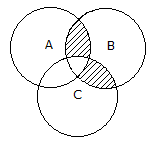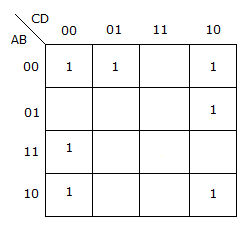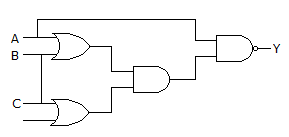# Electronics and Communication Engineering - Digital Electronics

11.

The boolean expression for shaded area in the given figure isA. AB + AC B. ABC + ABC C. ABC + ABC D. None of these

Explanation:

No answer description available for this question. Let us discuss.

12.

100101.10112 = __________ .

 A. 115.228 B. 115.548 C. 215.548 D. 100.018

Explanation:

No answer description available for this question. Let us discuss.

13.

The given figure shows a K-map for a Boolean function. The number of essential prime implicants isA. 4 B. 5 C. 6 D. 8

Explanation:

No answer description available for this question. Let us discuss.

14.

For the logic circuit given, what is the simplified Boolean function?A. X = AB + C B. X = BC + A C. X = AB + AC D. X = AC + B

Explanation:

No answer description available for this question. Let us discuss.

15.

An XOR gate with 6 variables is as follows A ⊕ B ⊕ C ⊕ D ⊕ E ⊕ F. The number of minterms in the Boolean expression is

 A. 6 B. 12 C. 64 D. 32